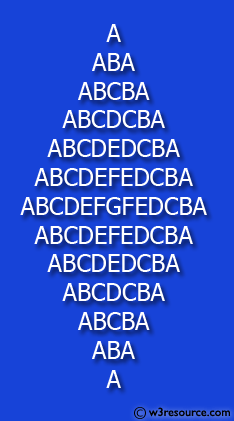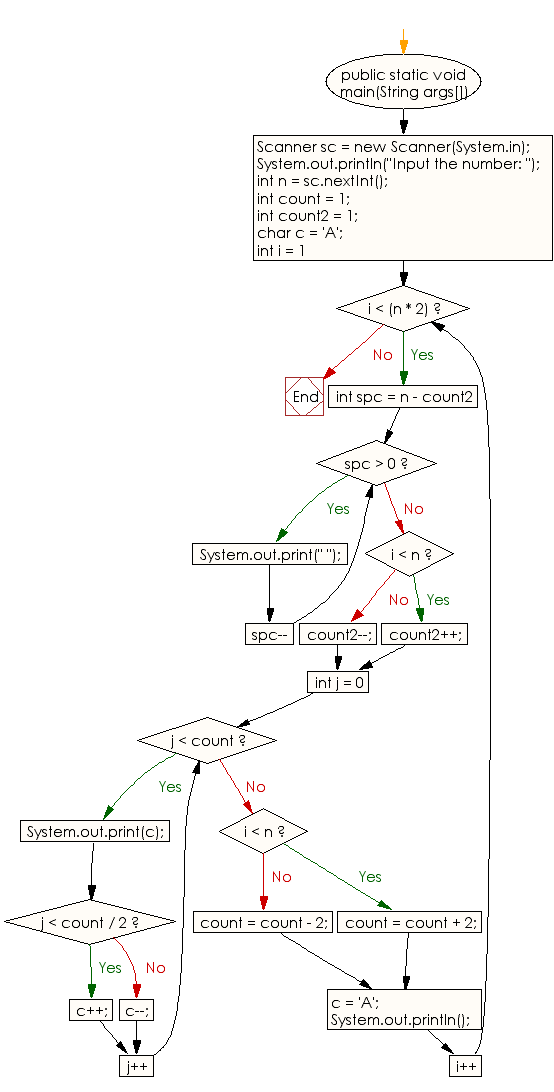﻿ Java exercises: Display the following character rhombus structure - w3resource# Java Conditional Statement Exercises: Display the following character rhombus structure

## Java Conditional Statement: Exercise-26 with Solution

Write a Java program to display the following character rhombus structure.

Test Data
Input the number: 7

Pictorial Presentation:Sample Solution:

Java Code:

``````import java.util.Scanner;
public class Exercise26 {
public static void main(String args[])
{
Scanner sc = new Scanner(System.in);
System.out.println("Input the number: ");
int n = sc.nextInt();
int count = 1;
int count2 = 1;
char c = 'A';

for (int i = 1; i < (n * 2); i++)
{
for (int spc = n - count2; spc > 0; spc--)
//print space
{
System.out.print(" ");
}
if (i < n)
{
count2++;
}
else
{
count2--;
}
for (int j = 0; j < count; j++)
{
System.out.print(c);//print Character
if (j < count / 2)
{
c++;
} else
{
c--;
}
}
if (i < n)
{
count = count + 2;
}
else
{
count = count - 2;
}
c = 'A';

System.out.println();
}
}
}
```
```

Sample Output:

```Input the number:
7
A
ABA
ABCBA
ABCDCBA
ABCDEDCBA
ABCDEFEDCBA
ABCDEFGFEDCBA
ABCDEFEDCBA
ABCDEDCBA
ABCDCBA
ABCBA
ABA
A
```

Flowchart:Java Code Editor: